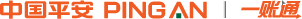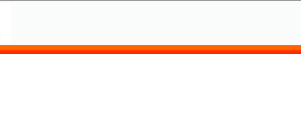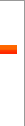# 重量容积换算

 蒲尔式 = 磅 蒲尔式=公吨= 长吨=短吨
 蒲尔式 = 磅 蒲尔式=公吨= 长吨=短吨
 蒲尔式 = 磅 蒲尔式=公吨= 长吨=短吨
 蒲尔式 = 磅 蒲尔式=公吨= 长吨=短吨
 短吨 = 磅 短吨=公吨
 英担 = 磅 英担=公吨
 蒲式耳 = 磅 蒲式耳=公吨
 磅 = 公吨 包=磅
 磅 = 公吨
 磅 = 公吨
 吨 = 桶